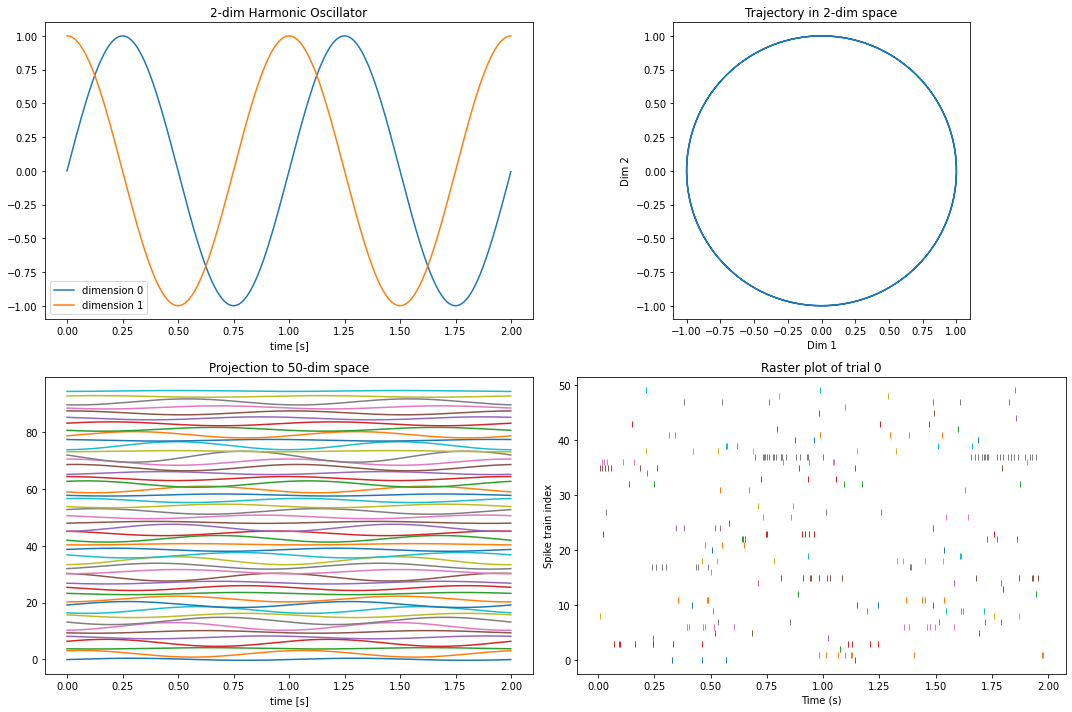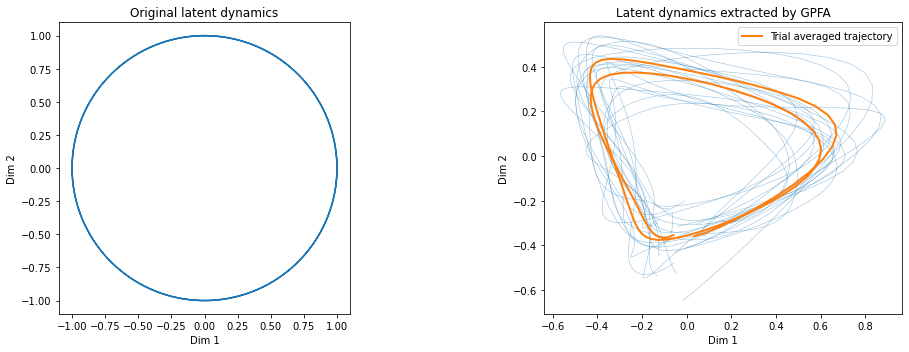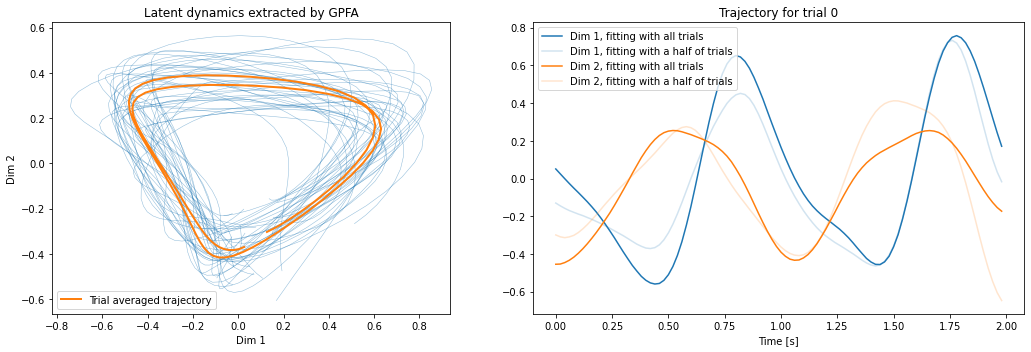# Tutorial: GPFA (Gaussian Process Factor Analysis)¶

Gaussian-process factor analysis (GPFA) is a dimensionality reduction method  for neural trajectory visualization of parallel spike trains. GPFA applies factor analysis (FA) to time-binned spike count data to reduce the dimensionality and at the same time smoothes the resulting low-dimensional trajectories by fitting a Gaussian process (GP) model to them.

The input consists of a set of trials ($Y$), each containing a list of spike trains (N neurons). The output is the projection ($X$) of the data in a space of pre-chosen dimensionality $x_{dim} < N$.

Under the assumption of a linear relation (transform matrix $C$) between the latent variable $X$ following a Gaussian process and the spike train data $Y$ with a bias $d$ and a noise term of zero mean and (co)variance $R$ (i.e., $Y = C X + d + \mathcal{N}(0,R)$), the projection corresponds to the conditional probability $E[X|Y]$. The parameters $(C, d, R)$ as well as the time scales and variances of the Gaussian process are estimated from the data using an expectation-maximization (EM) algorithm.

Internally, the analysis consists of the following steps:

1. bin the spike train data to get a sequence of $N$ dimensional vectors of spike counts in respective time bins, and choose the reduced dimensionality $x_{dim}$

2. expectation-maximization for fitting of the parameters $C, d, R$ and the time-scales and variances of the Gaussian process, using all the trials provided as input (c.f., gpfa_core.em())

3. projection of single trials in the low dimensional space (c.f., gpfa_core.exact_inference_with_ll())

4. orthonormalization of the matrix $C$ and the corresponding subspace, for visualization purposes: (c.f., gpfa_core.orthonormalize())

## 1. Idea of This Tutorial¶

This tutorial illustrates the usage of the gpfa.GPFA() class implemented in elephant, through its applications to synthetic spike train data, of which the ground truth low-dimensional structure is known.

The examples were inspired by the supplementary material of 

### 1.1. Generation of synthetic spike trains¶

A set of spike trains are generated as follows. First, a time series of either a 2-dimensional harmonic oscillator (Section 2) or a 3-dimensional Lorentz system (Section 3; the "standard" parameter values as seen in https://en.wikipedia.org/wiki/Lorenz_system are used) is projected into a high-dimensional space (as high dimension as the desired number of parallel spike trains) via a random projection. Then the resulting high-dimensional time series serves as time-dependent rates for an inhomogeneous multivariate Poisson process. Finally, multiple realizations of this Poisson process, which mimic spike trains from multiple trials, serve as input data to the GPFA.

Below are the functions used for spike train generation:

In [ ]:
import numpy as np
from scipy.integrate import odeint
import quantities as pq
import neo
from elephant.spike_train_generation import inhomogeneous_poisson_process

def integrated_oscillator(dt, num_steps, x0=0, y0=1, angular_frequency=2*np.pi*1e-3):
"""
Parameters
----------
dt : float
Integration time step in ms.
num_steps : int
Number of integration steps -> max_time = dt*(num_steps-1).
x0, y0 : float
Initial values in three dimensional space.
angular_frequency : float
Angular frequency in 1/ms.

Returns
-------
t : (num_steps) np.ndarray
Array of timepoints
(2, num_steps) np.ndarray
Integrated two-dimensional trajectory (x, y, z) of the harmonic oscillator
"""

assert isinstance(num_steps, int), "num_steps has to be integer"
t = dt*np.arange(num_steps)
x = x0*np.cos(angular_frequency*t) + y0*np.sin(angular_frequency*t)
y = -x0*np.sin(angular_frequency*t) + y0*np.cos(angular_frequency*t)
return t, np.array((x, y))

def integrated_lorenz(dt, num_steps, x0=0, y0=1, z0=1.05,
sigma=10, rho=28, beta=2.667, tau=1e3):
"""

Parameters
----------
dt :
Integration time step in ms.
num_steps : int
Number of integration steps -> max_time = dt*(num_steps-1).
x0, y0, z0 : float
Initial values in three dimensional space
sigma, rho, beta : float
Parameters defining the lorenz attractor
tau : characteristic timescale in ms

Returns
-------
t : (num_steps) np.ndarray
Array of timepoints
(3, num_steps) np.ndarray
Integrated three-dimensional trajectory (x, y, z) of the Lorenz attractor
"""
def _lorenz_ode(point_of_interest, timepoint, sigma, rho, beta, tau):
"""
Fit the model with spiketrains data and apply the dimensionality
reduction on spiketrains.

Parameters
----------
point_of_interest : tuple
Tupel containing coordinates (x,y,z) in three dimensional space.
timepoint : a point of interest in time
dt :
Integration time step in ms.
num_steps : int
Number of integration steps -> max_time = dt*(num_steps-1).
sigma, rho, beta : float
Parameters defining the lorenz attractor
tau : characteristic timescale in ms

Returns
-------
x_dot, y_dot, z_dot : float
Values of the lorenz attractor's partial derivatives
at the point x, y, z.
"""

x, y, z = point_of_interest

x_dot = (sigma*(y - x)) / tau
y_dot = (rho*x - y - x*z) / tau
z_dot = (x*y - beta*z) / tau
return x_dot, y_dot, z_dot

assert isinstance(num_steps, int), "num_steps has to be integer"

t = dt*np.arange(num_steps)
poi = (x0, y0, z0)
return t, odeint(_lorenz_ode, poi, t, args=(sigma, rho, beta, tau)).T

def random_projection(data, embedding_dimension, loc=0, scale=None):
"""
Parameters
----------
data : np.ndarray
Data to embed, shape=(M, N)
embedding_dimension : int
Embedding dimension, dimensionality of the space to project to.
loc : float or array_like of floats
Mean (“centre”) of the distribution.
scale : float or array_like of floats
Standard deviation (spread or “width”) of the distribution.

Returns
-------
np.ndarray
Random (normal) projection of input data, shape=(dim, N)

--------
np.random.normal()

"""
if scale is None:
scale = 1 / np.sqrt(data.shape)
projection_matrix = np.random.normal(loc, scale, (embedding_dimension, data.shape))
return np.dot(projection_matrix, data)

def generate_spiketrains(instantaneous_rates, num_trials, timestep):
"""
Parameters
----------
instantaneous_rates : np.ndarray
Array containing time series.
timestep :
Sample period.
num_steps : int
Number of timesteps -> max_time = timestep*(num_steps-1).

Returns
-------
spiketrains : list of neo.SpikeTrains
List containing spiketrains of inhomogeneous Poisson
processes based on given instantaneous rates.

"""

spiketrains = []
for _ in range(num_trials):
spiketrains_per_trial = []
for inst_rate in instantaneous_rates:
anasig_inst_rate = neo.AnalogSignal(inst_rate, sampling_rate=1/timestep, units=pq.Hz)
spiketrains_per_trial.append(inhomogeneous_poisson_process(anasig_inst_rate))
spiketrains.append(spiketrains_per_trial)

return spiketrains


## 2. Harmonic Oscillator Example¶

In this first example we apply the GPFA to spike train data derived from dynamics of a harmonic oscillator defined in a 2-dimensional latent variable space. The aim is to extract these 2-dimensional latent dynamics from the spike train data.

### 2.1. Generate synthetic spike train data¶

Here we generate 50-dimensional synthetic spike train data based on a trajectory of a 2-dimensional harmonic oscillator, as described in Section 1.1.

In [ ]:
# set parameters for the integration of the harmonic oscillator
timestep = 1 * pq.ms
trial_duration = 2 * pq.s
num_steps = int((trial_duration.rescale('ms')/timestep).magnitude)

# set parameters for spike train generation
max_rate = 70 * pq.Hz
np.random.seed(42)  # for visualization purposes, we want to get identical spike trains at any run

# specify data size
num_trials = 20
num_spiketrains = 50

# generate a low-dimensional trajectory
times_oscillator, oscillator_trajectory_2dim = integrated_oscillator(
timestep.magnitude, num_steps=num_steps, x0=0, y0=1)
times_oscillator = (times_oscillator*timestep.units).rescale('s')

# random projection to high-dimensional space
oscillator_trajectory_Ndim = random_projection(
oscillator_trajectory_2dim, embedding_dimension=num_spiketrains)

# convert to instantaneous rate for Poisson process
normed_traj = oscillator_trajectory_Ndim / oscillator_trajectory_Ndim.max()
instantaneous_rates_oscillator = np.power(max_rate.magnitude, normed_traj)

# generate spike trains
spiketrains_oscillator = generate_spiketrains(
instantaneous_rates_oscillator, num_trials, timestep)


Let's see how the trajectory and the spike trains look like.

In :
import matplotlib.pyplot as plt

f, ((ax1, ax2), (ax3, ax4)) = plt.subplots(2, 2, figsize=(15, 10))

ax1.set_title('2-dim Harmonic Oscillator')
ax1.set_xlabel('time [s]')
for i, y in enumerate(oscillator_trajectory_2dim):
ax1.plot(times_oscillator, y, label=f'dimension {i}')
ax1.legend()

ax2.set_title('Trajectory in 2-dim space')
ax2.set_xlabel('Dim 1')
ax2.set_ylabel('Dim 2')
ax2.set_aspect(1)
ax2.plot(oscillator_trajectory_2dim, oscillator_trajectory_2dim)

ax3.set_title(f'Projection to {num_spiketrains}-dim space')
ax3.set_xlabel('time [s]')
y_offset = oscillator_trajectory_Ndim.std() * 3
for i, y in enumerate(oscillator_trajectory_Ndim):
ax3.plot(times_oscillator, y + i*y_offset)

trial_to_plot = 0
ax4.set_title(f'Raster plot of trial {trial_to_plot}')
ax4.set_xlabel('Time (s)')
ax4.set_ylabel('Spike train index')
for i, spiketrain in enumerate(spiketrains_oscillator[trial_to_plot]):
ax4.plot(spiketrain, np.ones_like(spiketrain) * i, ls='', marker='|')

plt.tight_layout()
plt.show()Thus, we have generated 50-dimensional spike train data, derived from 2-dimensional latent dynamics, i.e., two cycles of circular rotation.

### 2.2. Apply GPFA to the generated data¶

Now we try to extract the original latent dynamics from the generated spike train data, by means of GPFA.

We first initialize an instance of the gpfa.GPFA() class. One can specify some parameters for model fitting at this timing. Here we set the size of the bin for spike train binning to 20 ms, and the dimensionality of latent variables to 2.

In [ ]:
from elephant.gpfa import GPFA

# specify fitting parameters
bin_size = 20 * pq.ms
latent_dimensionality = 2

gpfa_2dim = GPFA(bin_size=bin_size, x_dim=latent_dimensionality)


Then we call the fit() method of the class, with the generated spike train data as input. This fits a GPFA model to the given data, yielding estimates of the model parameters that best explain the data, which are stored in the params_estimated attribute of the class. Here we use the first half of the trials for fitting.

In [ ]:
gpfa_2dim.fit(spiketrains_oscillator[:num_trials//2])
print(gpfa_2dim.params_estimated.keys())


Then we transform the spike trains from the remaining half of the trials into tranjectories in the latent variable space, using the transform() method.

In [ ]:
trajectories = gpfa_2dim.transform(spiketrains_oscillator[num_trials//2:])


Let's see how the extracted trajectories look like.

In :
f, (ax1, ax2) = plt.subplots(1, 2, figsize=(15, 5))

linewidth_single_trial = 0.5
color_single_trial = 'C0'
alpha_single_trial = 0.5

linewidth_trial_average = 2
color_trial_average = 'C1'

ax1.set_title('Original latent dynamics')
ax1.set_xlabel('Dim 1')
ax1.set_ylabel('Dim 2')
ax1.set_aspect(1)
ax1.plot(oscillator_trajectory_2dim, oscillator_trajectory_2dim)

ax2.set_title('Latent dynamics extracted by GPFA')
ax2.set_xlabel('Dim 1')
ax2.set_ylabel('Dim 2')
ax2.set_aspect(1)
# single trial trajectories
for single_trial_trajectory in trajectories:
ax2.plot(single_trial_trajectory, single_trial_trajectory, '-', lw=linewidth_single_trial, c=color_single_trial, alpha=alpha_single_trial)
# trial averaged trajectory
average_trajectory = np.mean(trajectories, axis=0)
ax2.plot(average_trajectory, average_trajectory, '-', lw=linewidth_trial_average, c=color_trial_average, label='Trial averaged trajectory')
ax2.legend()

plt.tight_layout()
plt.show()GPFA successfuly exatracted, as the trial averaged trajectory, the two cycles of rotation in 2-dimensional latent space from the 50-dimensional spike train data.

In the above application we split the trials into two halves and performed fitting and transforming separately on these two sets of trials. One can also simply perform fitting and transforming on the whole dataset to obtain latent trajectories for all trials. In such a senario, the fit_transform() method can be used to perform the fitting and transforming at once, as shown in the example below.

In :
# here we just reuse the existing instance of the GPFA() class as we use the same fitting parameters as before
trajectories_all = gpfa_2dim.fit_transform(spiketrains_oscillator)

f, (ax1, ax2) = plt.subplots(1, 2, figsize=(15, 5))

ax1.set_title('Latent dynamics extracted by GPFA')
ax1.set_xlabel('Dim 1')
ax1.set_ylabel('Dim 2')
ax1.set_aspect(1)
for single_trial_trajectory in trajectories_all:
ax1.plot(single_trial_trajectory, single_trial_trajectory, '-', lw=linewidth_single_trial, c=color_single_trial, alpha=alpha_single_trial)
average_trajectory = np.mean(trajectories_all, axis=0)
ax1.plot(average_trajectory, average_trajectory, '-', lw=linewidth_trial_average, c=color_trial_average, label='Trial averaged trajectory')
ax1.legend()

trial_to_plot = 0
ax2.set_title(f'Trajectory for trial {trial_to_plot}')
ax2.set_xlabel('Time [s]')
times_trajectory = np.arange(len(trajectories_all[trial_to_plot])) * bin_size.rescale('s')
ax2.plot(times_trajectory, trajectories_all, c='C0', label="Dim 1, fitting with all trials")
ax2.plot(times_trajectory, trajectories, c='C0', alpha=0.2, label="Dim 1, fitting with a half of trials")
ax2.plot(times_trajectory, trajectories_all, c='C1', label="Dim 2, fitting with all trials")
ax2.plot(times_trajectory, trajectories, c='C1', alpha=0.2, label="Dim 2, fitting with a half of trials")
ax2.legend()

plt.tight_layout()
plt.show()

Initializing parameters using factor analysis...

Fitting GPFA model...We obtain almost the same latent dynamics, but single trial trajectories are slightly modified owing to an increased amount of the data used for fitting.

## 3. Lorentz System Example¶

### 3.1. Generate sysnthetic spike train data¶

Now we move on to the next example. Here we generate 50-dimensional synthetic spike train data based on a trajectory of a 3-dimensional Lorentz system. Note that, as we want to adopt a part of the trajectory where the double-wing structure of the attractor is fully developed as "trial", we drop off an initial part of the trajectory as "transient".

In [ ]:
# set parameters for the integration of the Lorentz attractor
timestep = 1 * pq.ms
transient_duration = 10 * pq.s
trial_duration = 30 * pq.s
num_steps_transient = int((transient_duration.rescale('ms')/timestep).magnitude)
num_steps = int((trial_duration.rescale('ms')/timestep).magnitude)

# set parameters for spike train generation
max_rate = 70 * pq.Hz
np.random.seed(42)  # for visualization purposes, we want to get identical spike trains at any run

# specify data
num_trials = 20
num_spiketrains = 50

# calculate the oscillator
times, lorentz_trajectory_3dim = integrated_lorenz(
timestep, num_steps=num_steps_transient+num_steps, x0=0, y0=1, z0=1.25)
times = (times - transient_duration).rescale('s').magnitude
times_trial = times[num_steps_transient:]

# random projection
lorentz_trajectory_Ndim = random_projection(
lorentz_trajectory_3dim[:, num_steps_transient:], embedding_dimension=num_spiketrains)

# calculate instantaneous rate
normed_traj = lorentz_trajectory_Ndim / lorentz_trajectory_Ndim.max()
instantaneous_rates_lorentz = np.power(max_rate.magnitude, normed_traj)

# generate spiketrains
spiketrains_lorentz = generate_spiketrains(
instantaneous_rates_lorentz, num_trials, timestep)


Let's plot the obtained trajectory and the spike trains.

In :
from mpl_toolkits.mplot3d import Axes3D

f = plt.figure(figsize=(15, 10))
ax1 = f.add_subplot(2, 2, 1)
ax2 = f.add_subplot(2, 2, 2, projection='3d')
ax3 = f.add_subplot(2, 2, 3)
ax4 = f.add_subplot(2, 2, 4)

ax1.set_title('Lorentz system')
ax1.set_xlabel('Time [s]')
labels = ['x', 'y', 'z']
for i, x in enumerate(lorentz_trajectory_3dim):
ax1.plot(times, x, label=labels[i])
ax1.axvspan(-transient_duration.rescale('s').magnitude, 0, color='gray', alpha=0.1)
ax1.text(-5, -20, 'Initial transient', ha='center')
ax1.legend()

ax2.set_title(f'Trajectory in 3-dim space')
ax2.set_xlabel('x')
ax2.set_ylabel('y')
ax2.set_ylabel('z')
ax2.plot(lorentz_trajectory_3dim[0, :num_steps_transient],
lorentz_trajectory_3dim[1, :num_steps_transient],
lorentz_trajectory_3dim[2, :num_steps_transient], c='C0', alpha=0.3)
ax2.plot(lorentz_trajectory_3dim[0, num_steps_transient:],
lorentz_trajectory_3dim[1, num_steps_transient:],
lorentz_trajectory_3dim[2, num_steps_transient:], c='C0')

ax3.set_title(f'Projection to {num_spiketrains}-dim space')
ax3.set_xlabel('Time [s]')
y_offset = lorentz_trajectory_Ndim.std() * 3
for i, y in enumerate(lorentz_trajectory_Ndim):
ax3.plot(times_trial, y + i*y_offset)

trial_to_plot = 0
ax4.set_title(f'Raster plot of trial {trial_to_plot}')
ax4.set_xlabel('Time (s)')
ax4.set_ylabel('Neuron id')
for i, spiketrain in enumerate(spiketrains_lorentz[trial_to_plot]):
ax4.plot(spiketrain, np.ones(len(spiketrain)) * i, ls='', marker='|')

plt.tight_layout()
plt.show()